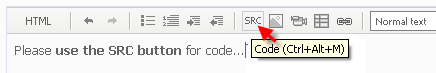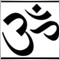# plzz convert this indicator to expert adviser162

hi,

am new to expert adversor trading,

i have 1 indicator that is 2 sma cross,

i need to change in expert advisor,

i dont know how to change.and how to write code with required parameters.

plzz help me.

thanks

```
#property indicator_separate_window
#property indicator_buffers 2
#property indicator_color1 Green
#property indicator_color2 DarkViolet
//---- input parameters
extern int x_prd=0;
extern int t3_period=8;
extern double b=0.7;
extern int CountBars=300;
//---- buffers
double t3[];
double prise[];
//+------------------------------------------------------------------+
//| Custom indicator initialization function                         |
//+------------------------------------------------------------------+
int init()
{
//---- indicator line
IndicatorBuffers(2);
SetIndexBuffer(0,t3);
SetIndexBuffer(1,prise);
SetIndexStyle(0,DRAW_HISTOGRAM);
SetIndexStyle(1,DRAW_LINE);

//----

//----
return(0);
}
//+------------------------------------------------------------------+
//| T3_DPO-v1                                                         |
//+------------------------------------------------------------------+
int start()
{
SetIndexDrawBegin(0,Bars-CountBars+t3_period+1);
SetIndexDrawBegin(1,Bars-CountBars+t3_period+1);
int i,counted_bars=IndicatorCounted();
double e1,e2,e3,e4,e5,e6,c1,c2,c3,c4,n,w1,w2, b2,b3,dpo,Prise;
//----
if(Bars<=t3_period) return(0);
//---- initial zero
if(counted_bars<t3_period)
{
for(i=1;i<=t3_period;i++) prise[Bars-i]=0.0;
for(i=1;i<=t3_period;i++) t3[Bars-i]=0.0;
}
//----
b2=b*b;
b3=b2*b;
c1=-b3;
c2=(3*(b2+b3));
c3=-3*(2*b2+b+b3);
c4=(1+3*b+b3+3*b2);
n=t3_period;

if (n<1) n=1;
n = 1 + 0.5*(n-1);
w1 = 2 / (n + 1);
w2 = 1 - w1;
//----
i=Bars-CountBars-1;
if(counted_bars>=t3_period) i=CountBars-counted_bars-1;
while(i>=0)
{
dpo=iMA(NULL,0,t3_period,0,MODE_SMA,PRICE_CLOSE,i);

e1 = w1*dpo + w2*e1;
e2 = w1*e1 + w2*e2;
e3 = w1*e2 + w2*e3;
e4 = w1*e3 + w2*e4;
e5 = w1*e4 + w2*e5;
e6 = w1*e5 + w2*e6;

t3[i] = c1*e6 + c2*e5 + c3*e4 + c4*e3;

prise[i]=Close[i];

i--;
}
return(0);
}
//+------------------------------------------------------------------+
```24692

vssshiva:

i have 1 indicator that is 2 sma cross,

i need to change in expert advisor,

No you don't. Use iCustom to get the values

Use SRC to post code5300

Does anyone know how he got the code in a Frame like that?17375

Like this I guess . . . .

```I did a copy paste of his HTML . . .

```5300

Thanks, I much prefer this format for lengthy-code posts. Tho 1 could attach a file, this would be helpful for at-a-glance helpers.